# Detection of peaks in data¶

Marcos Duarte
Laboratory of Biomechanics and Motor Control](http://demotu.org/
Federal University of ABC, Brazil

One way to detect peaks (local maxima) or valleys (local minima) in data is to use the property that a peak (or valley) must be greater (or smaller) than its immediate neighbors. The function detect_peaks.py from Python module detecta detects peaks (or valleys) based on this feature and other characteristics. The function signature is:

ind = detect_peaks(x, mph=None, mpd=1, threshold=0, edge='rising', kpsh=False, valley=False, show=False, ax=None, title=True)


The parameters mph, mpd, and threshold follow the convention of the Matlab function findpeaks.m.
Let's see how to use detect_peaks.py; first let's import the necessary Python libraries and configure the environment:

## Installation¶

pip install detecta


Or

conda install -c duartexyz detecta

In :
import numpy as np
import matplotlib.pyplot as plt
%matplotlib inline

from detecta import detect_peaks


Running the function examples:

In :
    >>> x = np.random.randn(100)
>>> x[60:81] = np.nan
>>> # detect all peaks and plot data
>>> ind = detect_peaks(x, show=True)
>>> print(ind)

>>> x = np.sin(2*np.pi*5*np.linspace(0, 1, 200)) + np.random.randn(200)/5
>>> # set minimum peak height = 0 and minimum peak distance = 20
>>> detect_peaks(x, mph=0, mpd=20, show=True)

>>> x = [0, 1, 0, 2, 0, 3, 0, 2, 0, 1, 0]
>>> # set minimum peak distance = 2
>>> detect_peaks(x, mpd=2, show=True)

>>> x = np.sin(2*np.pi*5*np.linspace(0, 1, 200)) + np.random.randn(200)/5
>>> # detection of valleys instead of peaks
>>> detect_peaks(x, mph=-1.2, mpd=20, valley=True, show=True)

>>> x = [0, 1, 1, 0, 1, 1, 0]
>>> # detect both edges
>>> detect_peaks(x, edge='both', show=True)

>>> x = [-2, 1, -2, 2, 1, 1, 3, 0]
>>> # set threshold = 2
>>> detect_peaks(x, threshold = 2, show=True)

>>> x = [-2, 1, -2, 2, 1, 1, 3, 0]
>>> fig, axs = plt.subplots(ncols=2, nrows=1, figsize=(10, 4))
>>> detect_peaks(x, show=True, ax=axs, threshold=0.5, title=False)
>>> detect_peaks(x, show=True, ax=axs, threshold=1.5, title=False)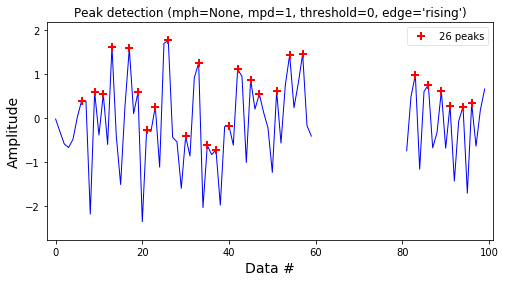[ 6  9 11 13 17 19 21 23 26 30 33 35 37 40 42 45 47 51 54 57 83 86 89 91
94 96]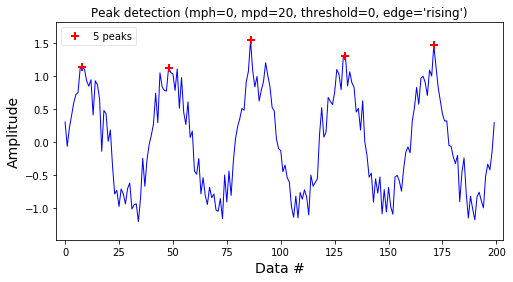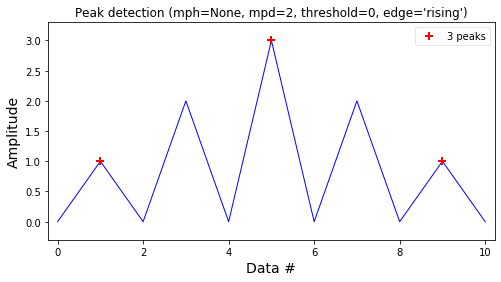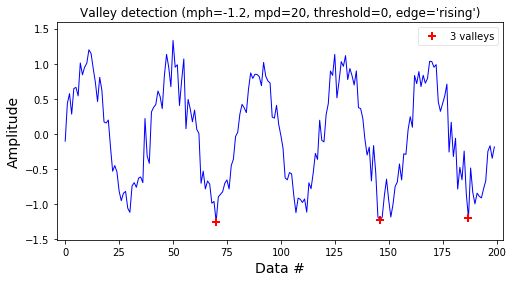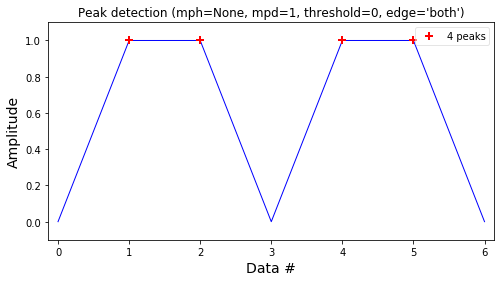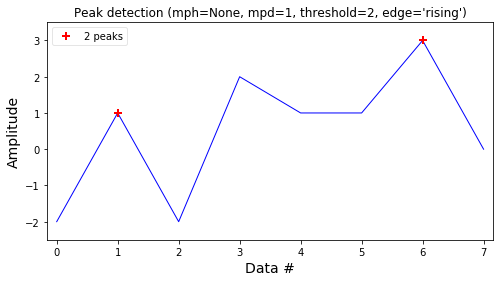Out:
array([1, 6])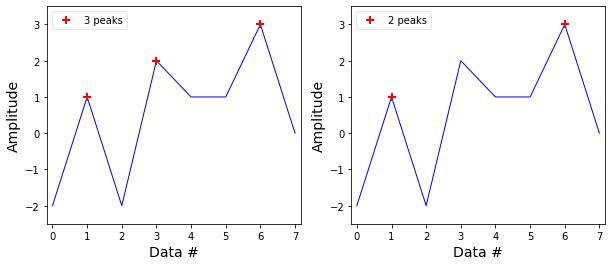## Function performance¶

The function detect_peaks.py is relatively fast but the parameter minimum peak distance (mpd) slows down the function if the data has several peaks (>1000). Try to decrease the number of peaks by tuning the other parameters or smooth the data before calling this function with several peaks in the data.
Here is a simple test of its performance:

In :
x = np.random.randn(10000)
ind = detect_peaks(x)
print('Data with %d points and %d peaks\n' %(x.size, ind.size))
print('Performance (without the minimum peak distance parameter):')
print('detect_peaks(x)')
%timeit detect_peaks(x)
print('\nPerformance (using the minimum peak distance parameter):')
print('detect_peaks(x, mpd=10)')
%timeit detect_peaks(x, mpd=10)

Data with 10000 points and 3358 peaks

Performance (without the minimum peak distance parameter):
detect_peaks(x)
98.2 µs ± 1.95 µs per loop (mean ± std. dev. of 7 runs, 10000 loops each)

Performance (using the minimum peak distance parameter):
detect_peaks(x, mpd=10)
8.17 ms ± 105 µs per loop (mean ± std. dev. of 7 runs, 100 loops each)